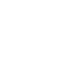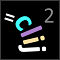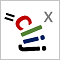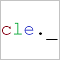# CLIJ2GPU accelerated image processing for everyone

CLIJ2 home

## differenceOfGaussian3DApplies Gaussian blur to the input image twice with different sigma values resulting in two images which are then subtracted from each other.

It is recommended to apply this operation to images of type Float (32 bit) as results might be negative.

### Parameters

input : Image The input image to be processed. destination : Image The output image where results are written into. sigma1_x : float Sigma of the first Gaussian filter in x sigma1_y : float Sigma of the first Gaussian filter in y sigma1_z : float Sigma of the first Gaussian filter in z sigma2_x : float Sigma of the second Gaussian filter in x sigma2_y : float Sigma of the second Gaussian filter in y sigma2_z : float Sigma of the second Gaussian filter in z

Categories: Filter, Noise, Background

Availability: Available in Fiji by activating the update sites clij and clij2. This function is part of clij2_-2.5.0.1.jar.

### Usage in ImageJ macro

``````Ext.CLIJ2_differenceOfGaussian3D(Image input, Image destination, Number sigma1x, Number sigma1y, Number sigma1z, Number sigma2x, Number sigma2y, Number sigma2z);
``````

### Usage in object oriented programming languages

Java
```// init CLIJ and GPU
import net.haesleinhuepf.clij2.CLIJ2;
import net.haesleinhuepf.clij.clearcl.ClearCLBuffer;
CLIJ2 clij2 = CLIJ2.getInstance();

// get input parameters
ClearCLBuffer input = clij2.push(inputImagePlus);
destination = clij2.create(input);
float sigma1x = 1.0;
float sigma1y = 2.0;
float sigma1z = 3.0;
float sigma2x = 4.0;
float sigma2y = 5.0;
float sigma2z = 6.0;
```
```// Execute operation on GPU
clij2.differenceOfGaussian3D(input, destination, sigma1x, sigma1y, sigma1z, sigma2x, sigma2y, sigma2z);
```
```// show result
destinationImagePlus = clij2.pull(destination);
destinationImagePlus.show();

// cleanup memory on GPU
clij2.release(input);
clij2.release(destination);
```
Matlab
```% init CLIJ and GPU
clij2 = init_clatlab();

% get input parameters
input = clij2.pushMat(input_matrix);
destination = clij2.create(input);
sigma1x = 1.0;
sigma1y = 2.0;
sigma1z = 3.0;
sigma2x = 4.0;
sigma2y = 5.0;
sigma2z = 6.0;
```
```% Execute operation on GPU
clij2.differenceOfGaussian3D(input, destination, sigma1x, sigma1y, sigma1z, sigma2x, sigma2y, sigma2z);
```
```% show result
destination = clij2.pullMat(destination)

% cleanup memory on GPU
clij2.release(input);
clij2.release(destination);
```
Icy JavaScript
```// init CLIJ and GPU
importClass(net.haesleinhuepf.clicy.CLICY);
importClass(Packages.icy.main.Icy);

clij2 = CLICY.getInstance();

// get input parameters
input_sequence = getSequence();
input = clij2.pushSequence(input_sequence);
destination = clij2.create(input);
sigma1x = 1.0;
sigma1y = 2.0;
sigma1z = 3.0;
sigma2x = 4.0;
sigma2y = 5.0;
sigma2z = 6.0;
```
```// Execute operation on GPU
clij2.differenceOfGaussian3D(input, destination, sigma1x, sigma1y, sigma1z, sigma2x, sigma2y, sigma2z);
```
```// show result
destination_sequence = clij2.pullSequence(destination)
// cleanup memory on GPU
clij2.release(input);
clij2.release(destination);
```
clEsperanto Python (experimental)
```import pyclesperanto_prototype as cle

cle.difference_of_gaussian(input, destination, sigma1x, sigma1y, sigma1z, sigma2x, sigma2y, sigma2z)

```

Imprint